# Vlookup函数最常见错误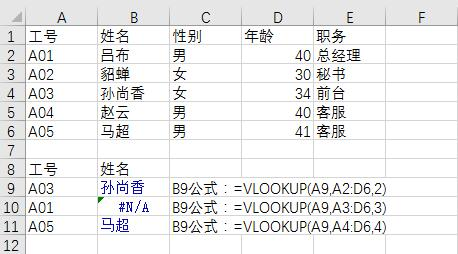B9公式改为：=VLOOKUP(A9,\$A\$2:\$D\$6,2,0）

2 在公式中用trim函数替换空格而必须要用数据公式形式输入。

{=VLOOKUP(A9,TRIM(A1:D6),2,0)}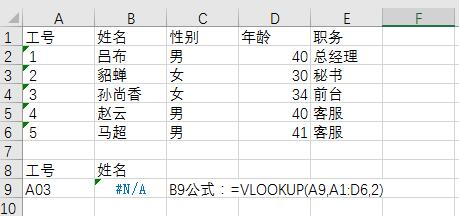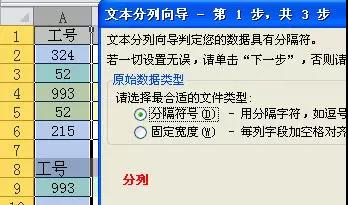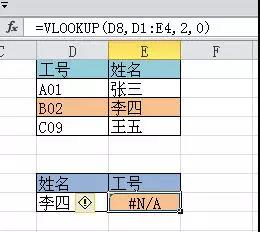1 用if函数重组区域，让两列颠倒位置。
=VLOOKUP(D8,IF({0,1},D2:D4,E2:E4),2,0)
2 用index+match组合实现。
=INDEX(D2:D4,MATCH(D8,E2:E4,0))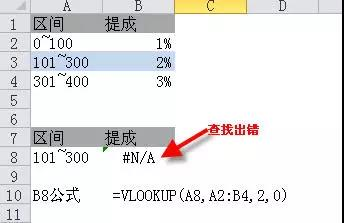=VLOOKUP(SUBSTITUTE(A8,"~","~~"),A2:B4,2,0)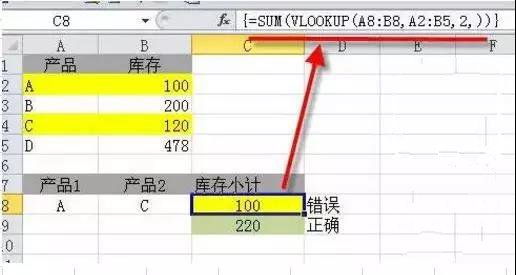=SUM(VLOOKUP(T(IF({1},A8:B8)),A2:B5,2,))